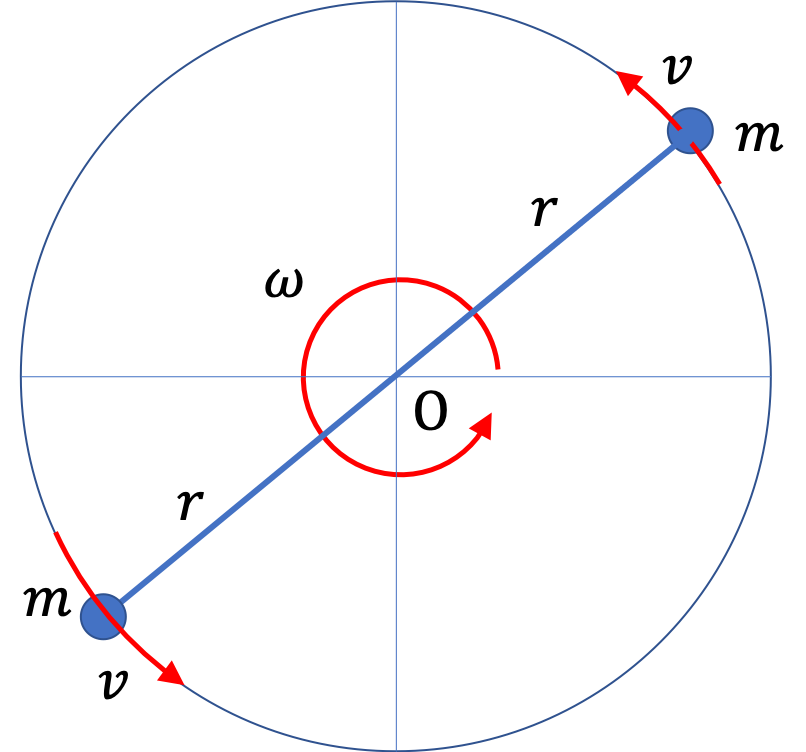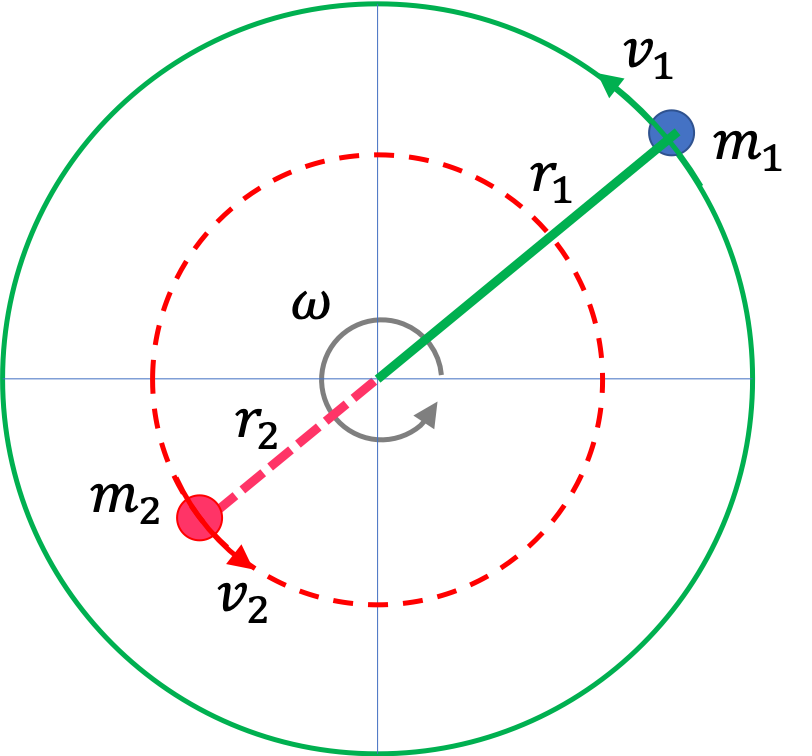## Section9.15Rotational Kinetic Energy

Recall that $\vec F_\text{net}=m\vec a$ implied a work-energy theorem on a path of motion. By following very similar steps, we can deduce a work-energy theorem for rotation from $\mathcal{T}=I\alpha\text{.}$

To do that let us examine rotational work done over an interval. The work by all torques on a system will be obtained by summing $\mathcal{T} \Delta\theta$ from the initial time to the final time for each torque.

\begin{equation} W_{if}^{\text{rot}} = \sum\, \mathcal{T} \Delta\theta.\label{rotational-kinetic-energy-1}\tag{9.15.1} \end{equation}

Now, recall that $\alpha$ in $\mathcal{T}=I\alpha$ is actually $\Delta\omega/\Delta t$ for infinitesimal $\Delta t\text{,}$ i.e., rate of change of angular velocity. Therefore, we write

\begin{equation} {\mathcal{T}}_{\text{net}} = I \, \alpha = I \, \dfrac{\Delta \omega}{\Delta t}.\label{rotational-kinetic-energy-2}\tag{9.15.2} \end{equation}

Using this $\mathcal{T}$ in Eq. (9.15.1) we get

\begin{equation*} W_{if}^{\text{rot}} = \sum\, I \, \dfrac{\Delta \omega}{\Delta t} \Delta\theta = \sum\, I \,\Delta \omega\, \dfrac{\Delta\theta}{\Delta t}= \sum\, I \,\Delta \omega\, \omega. \end{equation*}

(Calculus Step) The right hand side is a cumulative effect of infinitesimal intervals, which makes it an integral.

\begin{equation*} W_{if}^{\text{rot}} = \int_{\omega_i}^{\omega_f} I \, \omega\, d\omega. \end{equation*}

Carrying out the integral we get

\begin{equation} W_{if}^{\text{rot}} = \dfrac{1}{2}\, I\omega_f^2 - \dfrac{1}{2}\, I\omega_i^2.\label{rotational-kinetic-energy-half-I-omega-squared}\tag{9.15.3} \end{equation}

The quantity $\dfrac{1}{2} I\omega^2$ is rotational kinetic energy of the body. We will denote this by $K_{\text{rot}}\text{.}$

\begin{equation} K_{\text{rot}} = \dfrac{1}{2} I\omega^2.\tag{9.15.4} \end{equation}

Writing Eq. (9.15.3) in the following form gives us the result the net rotational work is the change in rotational kinetic energy.

\begin{equation} W_{if}^{\text{rot}} = K_{f}^{\text{rot}} - K_{i}^{\text{rot}}.\tag{9.15.5} \end{equation}

A baton has two balls at the end of a light beam of length $0.80\text{ m} \text{.}$ Let the masses of the two balls be $0.25\text{ kg}$ each. The baton is gently held at the center and rotated at an angular speed of $10\text{ rad/sec} \text{.}$ What is the kinetic energy of the baton? Ignore the mass of the beam.Hint

Use $I = m_1r_1^2 + m_2r_2^2\text{.}$

$4.0\text{ J}.$

Solution

Since the baton is in a pure rotation, we wil use the kinetic energy formula for the pure rotation.

\begin{align*} K \amp = \dfrac{1}{2}\, I\, \omega^2, \\ \amp = \dfrac{1}{2} (m_1r_1^2 + m_2r_2^2)\,\omega^2,\\ \amp = \dfrac{1}{2}\, 2\, m\, r^2\, \omega^2, \\ \amp = \dfrac{1}{2}\times 2\times 0.25 \times 0.4^2\times 10^2 = 4.0 \text{ J}. \end{align*}

A baton has two balls at the end of a light beam of length $0.80\text{ m} \text{.}$ Let the masses of the two balls be $0.25\text{ kg}$ each. The baton is gently held $0.20\text{ m}$ away from the center and rotated at an angular speed of $10\text{ rad/sec} \text{.}$ In a pure rotation, all parts of a rigid body have the same angular speed. What is the kinetic energy of the baton? Ignore the mass of the beam.Hint

Use $I = m_1r_1^2 + m_2r_2^2\text{.}$

$5.0\text{ J}.$

Solution

Since the baton is in a pure rotation, we wil use the kinetic energy formula for the pure rotation.

\begin{align*} K \amp = \dfrac{1}{2}\, I\, \omega^2, \\ \amp = \dfrac{1}{2} (m_1r_1^2 + m_2r_2^2)\,\omega^2,\\ \amp = \dfrac{1}{2} m (r_1^2 + r_2^2)\,\omega^2\\ \amp = \dfrac{1}{2} \times 0.25 \times ( 0.6^2 + 0.2^2)\times 10^2 = 5.0 \text{ J}. \end{align*}

A uniform density disk of mass $50\text{ kg}$ and radius $12\text{ cm}$ is set on an axle through its center and rotated from rest.

(a) How much work is required to bring the angular speed from zero to $10\text{ rev/sec}\text{?}$

(b) How much work is required to change the angular speed from $10\text{ rev/sec}$ to $20\text{ rev/sec}\text{?}$

(c) If an average force of $20\text{ N}$ acts at the rim in the direction of the tangent to the rim of the disk continuously, how many full rotations would it take to reach $10\text{ rev/sec}$ from rest?

(d) If you want to get to $20\text{ rev/sec}$ from $10\text{ rev/sec}$ in half as many rotations as it took you to get from rest to $10\text{ rev/sec}$ using the average $20\text{ N}$ force, what average force would you need to act along the tangent of the rim?

Hint

(a) Use Rot. KE formula, (b) Use $K_f-K_i\text{,}$ (c) Use work-energy theorem, (d) (d) Set up work energy theorem with required force as unknown.

(a) $711\text{ J} \text{,}$ (b) $2,132\text{ J}\text{,}$ (c) $47.15\text{ revs} \text{,}$ (d) $120\text{ N}\text{.}$

Solution 1 (a)

(a) The net rotational work needed will be equal to the change in the rotational kientic energy. For that, let us calculate the moment of inertia first. Using the formula for the disk we have

\begin{equation*} I = \dfrac{1}{2}\,MR^2 = \dfrac{1}{2}\times 50 \times 0.12^2 = 0.36\text{ kg.m}^2. \end{equation*}

The initial rotational speed is zero and the final rotational speed is

\begin{equation*} \omega_f = 10\times 2\pi = 20\pi\text{ rad/sec}, \end{equation*}

since there are $2\pi$ radians in each full cycle. Therefore, the change in rotational kinetic energy is

\begin{equation*} \Delta K_\text{rot} = \dfrac{1}{2}\times 0.36\times (20\pi)^2 - 0 = 711\text{ J}. \end{equation*}

This is the amount of work required.

Solution 2 (b)

(b) Now the change in rotational kinetic energy is different.

\begin{equation*} \Delta K_\text{rot} = \dfrac{1}{2}\times 0.36\times (40\pi)^2 - \dfrac{1}{2}\times 0.36\times (20\pi)^2 = 2,132\text{ J}. \end{equation*}
Solution 3 (c)

(c) From (a), we need to $711\text{ J}$ of work. With torque

\begin{equation*} \tau = 20\text{ N} \times 0.12\text{ m} = 2.40\text{ N.m}, \end{equation*}

acting or a rotation angle of $\Delta\theta$ will produce the required work by the work-energy theorem. That is,

\begin{equation*} 2.40\text{ N.m}\times \Delta\theta = 711\text{ J}. \end{equation*}

This gives the angle in radians.

\begin{equation*} \Delta \theta = 296.25\text{ rad}, \end{equation*}

which is

\begin{equation*} 296.25\text{ rad} = \dfrac{296}{2\pi}\text{ revs} = 47.15\text{ revs}. \end{equation*}
Solution 4 (d)

(d) From (b), we need to $2,132\text{ J}$ of work. But now in $296.25\text{ rad}/2$ displacement. Therefore, the required force must obly

\begin{equation*} F\times 0.12\text{ m} \times 296.25/2 \text{ rad} = 2,132 \text{ J}. \end{equation*}

Therefore,

\begin{equation*} F = 120\text{ N}. \end{equation*}